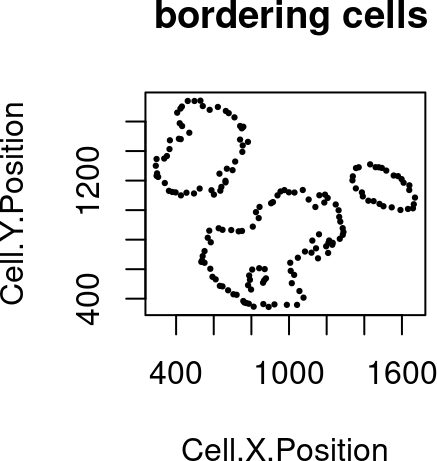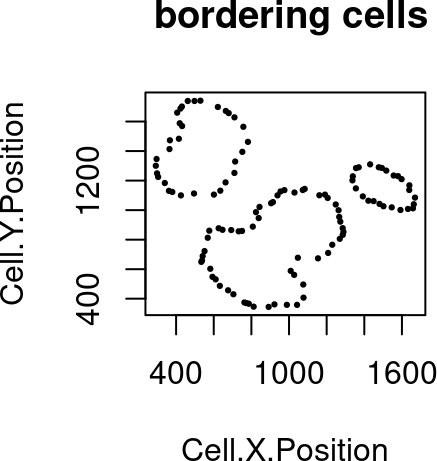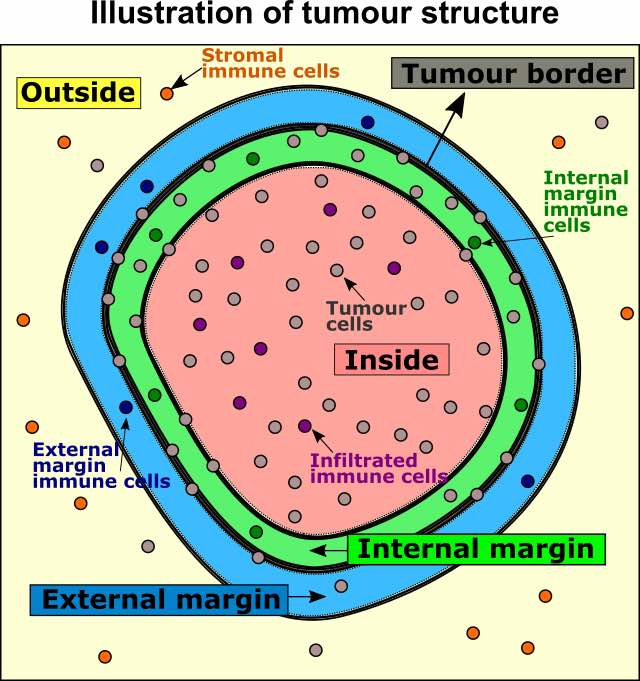``library(SPIAT)``

# 1 Characterising the distribution of the cells of interest in identified tissue regions

In certain analysis the focus is to understand the spatial distribution of a certain type of cell populations relative to the tissue regions.

One example of this functionality is to characterise the immune population in tumour structures. The following analysis will focus on the tumour/immune example, including determining whether there is a clear tumour margin, automatically identifying the tumour margin, and finally quantifying the proportion of immune populations relative to the margin. However, these analyses can also be generalised to other tissue and cell types.

In this vignette we will use an inForm data file that’s already been formatted for SPIAT with `format_image_to_spe()`, which we can load with `data()`. We will use `define_celltypes()` to define the cells with certain combinations of markers.

``````data("simulated_image")

# define cell types
formatted_image <- define_celltypes(
simulated_image,
categories = c("Tumour_marker","Immune_marker1,Immune_marker2",
"Immune_marker1,Immune_marker3",
"Immune_marker1,Immune_marker2,Immune_marker4", "OTHER"),
category_colname = "Phenotype",
names = c("Tumour", "Immune1", "Immune2", "Immune3", "Others"),
new_colname = "Cell.Type")``````

## 1.1 Determining whether there is a clear tumour margin

In some instances tumour cells are distributed in such a way that there are no clear tumour margins. While this can be derived intuitively in most cases, SPIAT offers a way of quantifying the ‘quality’ of the margin for downstream analyses. This is meant to be used to help flag images with relatively poor margins, and therefore we do not offer a cutoff value.

To determine if there is a clear tumour margin, SPIAT can calculate the ratio of tumour bordering cells to tumour total cells (R-BT). This ratio is high when there is a disproportional high number of tumour margin cells compared to internal tumour cells.

``R_BC(formatted_image, cell_type_of_interest = "Tumour", "Cell.Type")````##  0.2014652``

The result is 0.2014652. This low value means there are relatively low number of bordering cells compared to total tumour cells, meaning that this image has clear tumour margins.

## 1.2 Automatic identification of the tumour margin

We can identify margins with `identify_bordering_cells()`. This function leverages off the alpha hull method (Pateiro-Lopez, Rodriguez-Casal, and. 2019) from the alpha hull package. Here we use tumour cells (Tumour_marker) as the reference to identify the bordering cells but any cell type can be used.

``````formatted_border <- identify_bordering_cells(formatted_image,
reference_cell = "Tumour",
feature_colname="Cell.Type")``````
``##  "The alpha of Polygon is: 63.24375"````````# Get the number of tumour clusters
attr(formatted_border, "n_of_clusters")``````
``##  3``

There are 3 tumour clusters in the image.

## 1.3 Classification of cells based on their locations relative to the margin

We can then define four locations relative to the margin based on distances: “Internal margin”, “External margin”, “Outside” and “Inside”. Specifically, we define the area within a specified distance to the margin as either “Internal margin” (bordering the margin, inside the tumour area) and “External margin” (bordering the margin, surrounding the tumour area). The areas located further away than the specified distance from the margin are defined as “Inside” (i.e. the tumour area) and “Outside” (i.e. the tumour area).First, we calculate the distance of cells to the tumour margin.

``formatted_distance <- calculate_distance_to_margin(formatted_border)``
``````##  "Markers had been selected in minimum distance calculation: "
##  "Non-border" "Border"``````

Next, we classify cells based on their location. As a distance cutoff, we use a distance of 5 cells from the tumour margin. The function first calculates the average minimum distance between all pairs of nearest cells and then multiples this number by 5. Users can change the number of cell layers to increase/decrease the margin width.

``````names_of_immune_cells <- c("Immune1", "Immune2","Immune3")

formatted_structure <- define_structure(
formatted_distance, cell_types_of_interest = names_of_immune_cells,
feature_colname = "Cell.Type", n_margin_layers = 5)

categories <- unique(formatted_structure\$Structure)``````

We can plot and colour these structure categories.

``plot_cell_categories(formatted_structure, feature_colname = "Structure")``